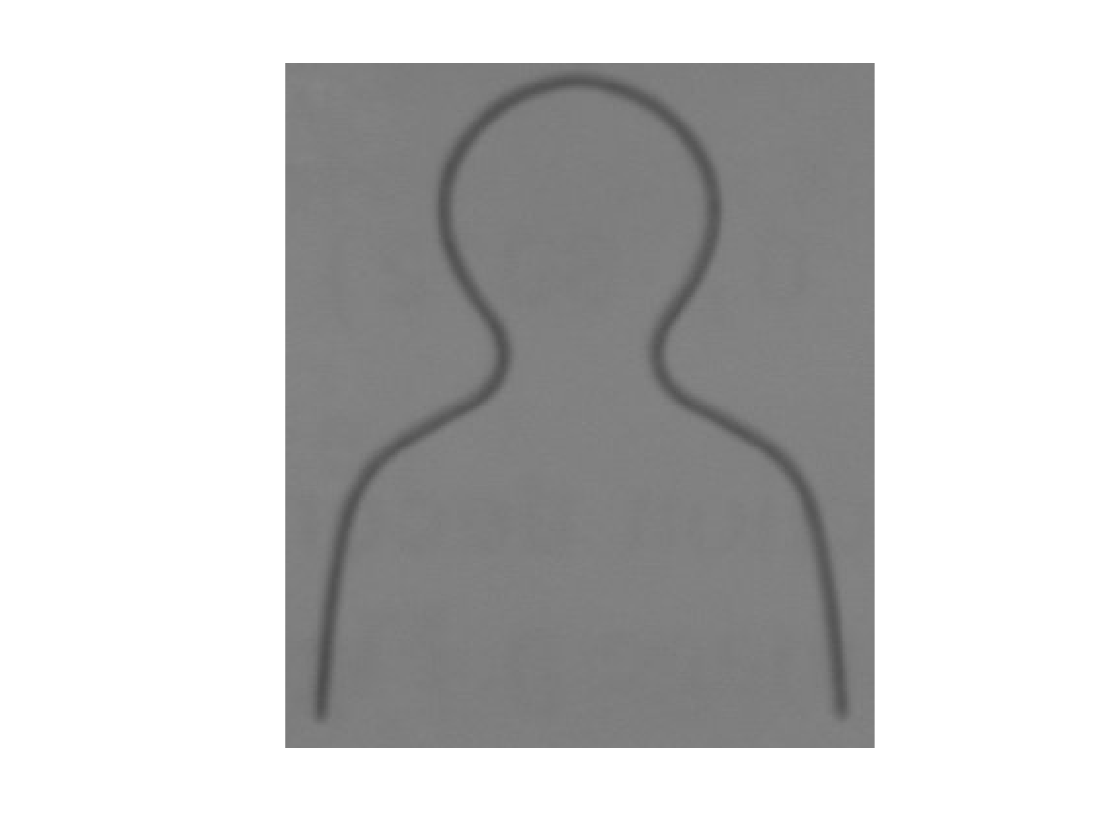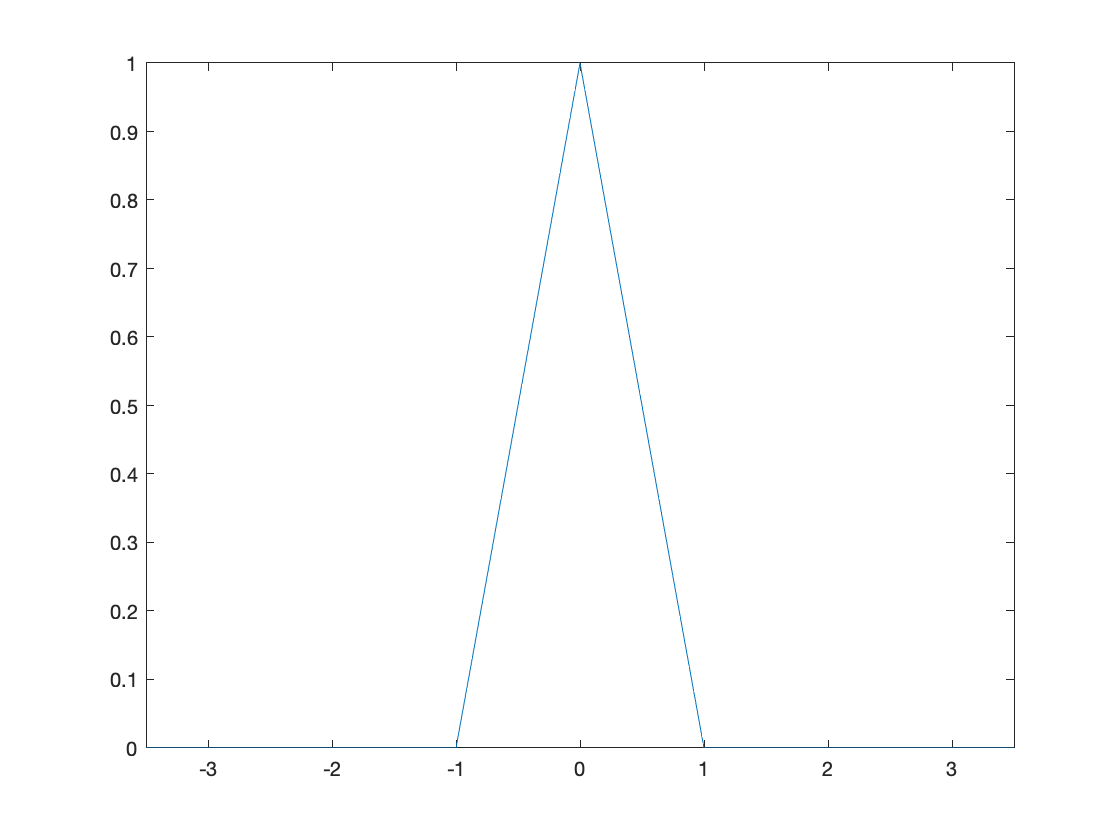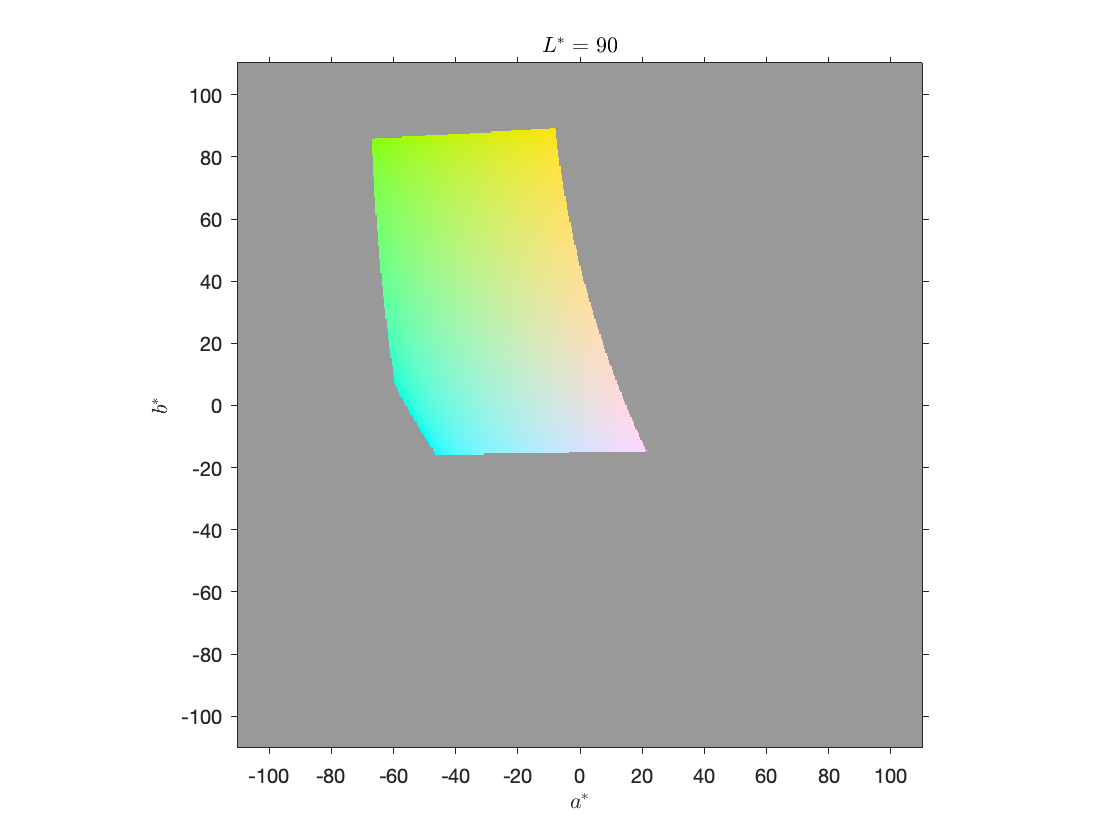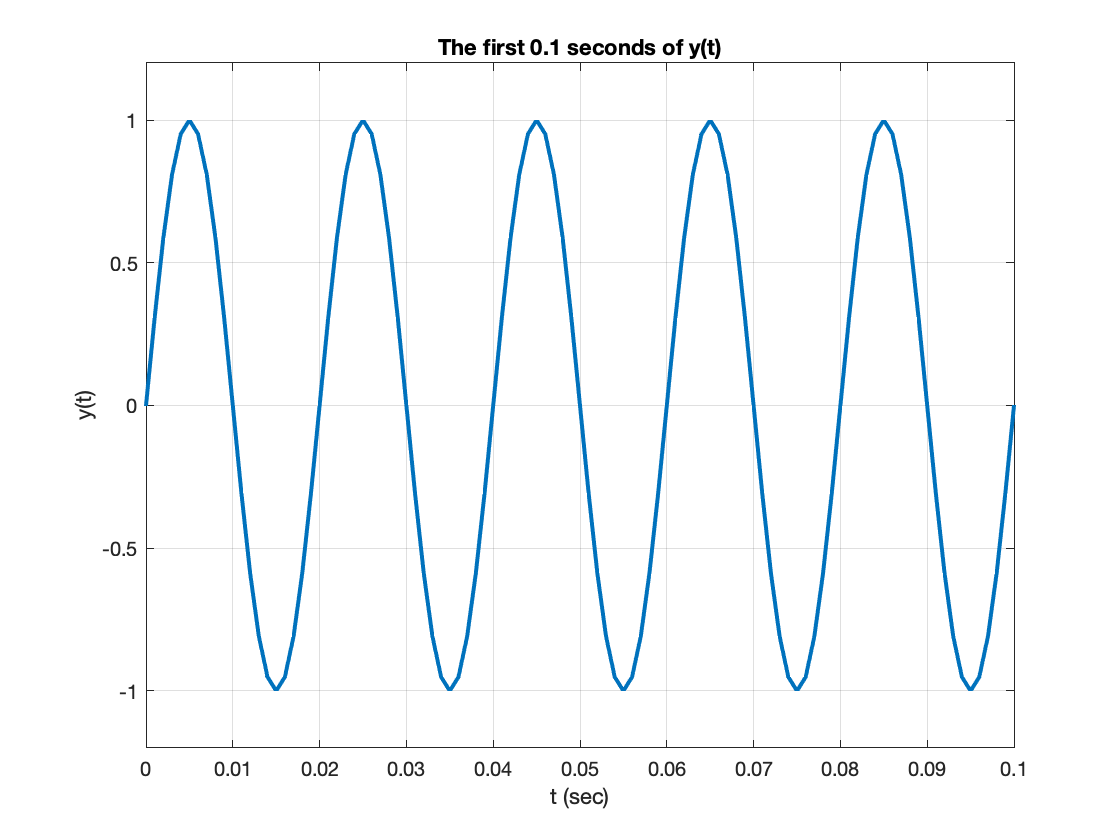Community Profile# Steve Eddins

### MathWorks

Last seen: 1 day ago Active since 2006

MATLAB user since 1987. MathWorker since 1993. Amateur French horn player. MATLAB design and development, image processing algorithms.

Image processing blog: https://blogs.mathworks.com/steve/

#### Statistics

All
••••••••••••#### Content Feed

View by

Can a MATLAB function edit the input?
You'll need your class to subclass handle. See the documentation page "Implementing Linked Lists with Classes" for details.

3 months ago | 0

| accepted

Matlab working very slow on my m1 MacBook Pro

5 months ago | 2

Shouldn't bwconvhull() be idempotent?
Hi Matt, At a general level - and speaking from too much personal experience - expectations about geometrical operations such a...

5 months ago | 2

| accepted

Issue in plotting binary image boundaries with real axis values
The function bwboundaries returns boundary values in pixel coordinates. You'll need to scale those to world coordinates yourself...

5 months ago | 0

| accepted

How to change boundary width in Active Contour Method?
Call visboundaries with an output argument. That output argument will be an hggroup object containing two lines. You can then ma...

5 months ago | 0

| accepted

Published

Use imbinarize to Threshold Gray-Scale Images
Today, I want to convince you to use imbinarize instead of im2bw.Background: I recently saw some data suggesting that many...

5 months agoPublished

NaN Sightings
Some of us at MathWorks like to share our "NaN sightings." Here is an example to show what I mean. This is a screenshot of...

6 months agoPublished

How to Use a Custom Interpolation Kernel with imresize
Recently, I was talking with MathWorks writer Jessica Bernier about the reference page for the imresize function. Jessica...

7 months agoPublished

How to Run Blog Post Code in Your Browser
I have published more than 560 blog posts here since 2006, and I estimate that about 98% of them started out as MATLAB...

7 months agoPublished

Plotting a* and b* colors
Today's blog post comes from planning one topic, but then taking a sharp left turn and doing something else completely. I...

7 months agoHaving graycomatrix rescaling problem
Here is the doc for the NumLevels parameter: Number of gray levels, specified as an integer. For example, if NumLevels is 8, gr...

8 months ago | 0

| accepted

How to find the center position under the saturation spot signal
Try converting the image to binary using imbinarize and then using regionprops to compute the centroid. rgb = imread('image.bmp...

8 months ago | 0

| accepted

How to bold x axis labels
Try setting the TickLabelsInterpreter property to 'tex', like this: plot(1:10) ax = gca; ax.XAxis.TickLabelInterpreter = 'tex...

9 months ago | 0

three difference vectors and plots
I have copy-pasted your code below, exactly as entered in your question. It runs and produces the plot with no error. I suggest ...

9 months ago | 2

Problem with datetime and daily values
Use a timetable and the retime function. >> T = readtable("data.xlsx"); >> head(T) ans = 8×5 table Date ...

9 months ago | 0

| accepted

What is the best way to count the occurrences of two table columns together?
In my previous answer, I forgot that grpstats is in the Statistics and Machine Learning Toolbox. Here is an answer using groupsu...

9 months ago | 1

What is the best way to count the occurrences of two table columns together?
I think grpstats will do what you want: >> grpstats(T,["A" "B"]) ans = 4×3 table A B Grou...

9 months ago | 0

Find peaks not working on my data set
I suspect the issue is with the x-coordinates that you are using to plot the peaks. You are plotting the peaks against the indic...

9 months ago | 1

| accepted

Assign values in a matrix
One idea is to use a timetable and the retime function. Suppose I have the following matrix for a 4-minute charge and a 3-minute...

9 months ago | 0

| accepted

Code is showing Undefined function 'pixelHull' for input arguments of type 'double'.
I wrote a function called pixelHull as part of my 16-Mar-2018 blog post. You can find the function listing near the bottom of th...

9 months ago | 0

| accepted

Published

FFT Spectral Leakage
A MATLAB user recently contacted MathWorks tech support to ask why the output of fft did not meet their expectations, and...

9 months agoBinarize image for specific colour
Use the Color Thresholder app to generate the code needed to do this segmentation. See my 14-Oct-2019 and 25-Apr-2014 blog posts...

10 months ago | 0

Plotting signal of a region of interest
A possible explanation is that you have a variable called "size" or "mean" in your workspace. For example, if you have a variabl...

10 months ago | 0

How to apply a threshold value to the cell array
I think writing a for-loop would be most straightforward way to go. Something like this: for k = 1:numel(C) C_k = C{k}; ...

10 months ago | 1

| accepted

How to change the font of a pie chart?
The text labels created by the pie function are not controlled by the font properties of the containing axes object. They are in...

10 months ago | 2

| accepted

superimpose matrices (n,n,m) to (n,n,1)
Here are some options. Compute and plot the maximum value at each pixel: imagesc(max(a100,[],3)) Compute and plot the minimum...

11 months ago | 0

| accepted

Image conversion from uint16 to uint8 mismatch
im2uint8 is using a scale factor of (255/65535), not 1/256. Note that scaling the maximum of the uint16 range, 65535, by 1/256 ...

11 months ago | 1

| accepted

How do I map array values on a logical array
Use logical indexing. C = zeros(size(B)); C(logical(B)) = A

11 months ago | 1

| accepted